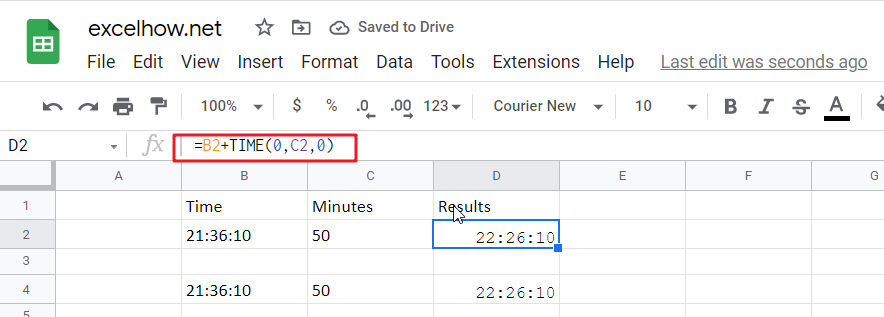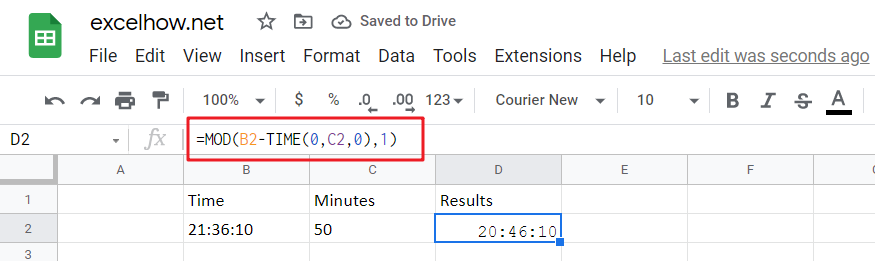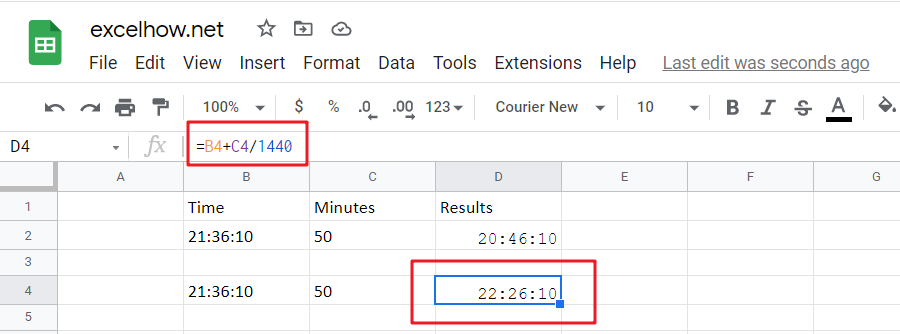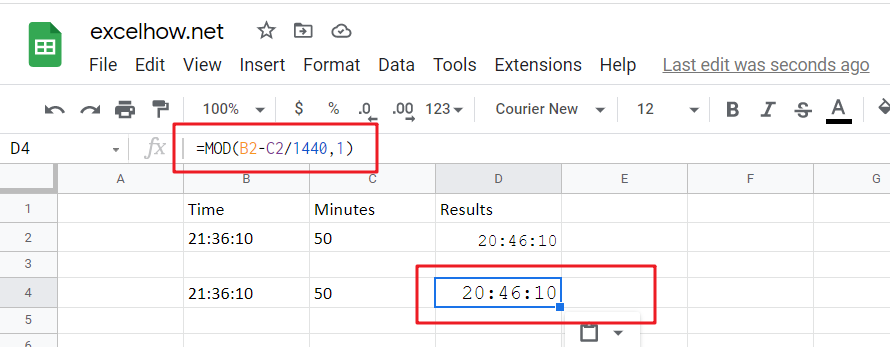Adding whole hours or decimal minutes to a given time in google sheets is a fairly simple process. You can do this by using the Start_time+TIME(0,minutes,0) and Start_time+minutes/1440 functions. In this add minutes to time guide, we will show you how to do this.If you want to add whole minutes to a given time, you can use the following formula:

`=Start_time+TIME(0, minutes,0)`
• Start_time: the time that you want to add minutes to
• Minutes: the minutes that you wish to add

This function returns a time formatted string.

Cell C2 shows the number of minutes you want to add, while Cell D2 displays a formula that will calculate how many more cells need changing.

`=B2+TIME(0,C2,0)`

Or

`=B4+C4/1440`

To see the result, press Enter key.### Explanation

The TIME function is a great way to convert decimal numbers into an appropriate time format. Here, we change the number of minutes from 50 (which converts it into PM) and then add:00 before adding another set amount for minutes-in, in this case, 0 because there aren’t any other digits available on our keyboard!

Note: To subtract the minutes from a time period, you need to use this formula.

`= MOD(Start_time-TIME(0, minutes,0),1)`

For Instance, Cell B2 shows time, and cell C2 shows the number of minutes you want to subtract. If you want to subtract a number of minutes, use the below formula:

`=MOD(B2-TIME(0,C2,0),1)`

To see the result, hit Enter key.The MOD (modulus) function is an important tool for financial professionals. It can be used to flip negative numbers into positive ones yield accurate results in most cases!

## Add decimal Minutes to time

Generic formula:

`=Start_time+ minutes /1440`
• Start_time: the time that you wish to add minutes to.
• minutes: the minutes that you wish to add to start_time.

The value should be returned in time format.

For instance, cell B2 contains the time value, and cell C2 displays the number of minutes you want to add. Please see the below formula for this purpose.

`=B2+C2/1440`

To see the result hit Enter key.24 hours in a day, and one minutes is 1/1440th of the whole duration.

Note: Subtracting the minutes from time is easy with this formula.

`=MOD(Start_time- minutes /1440,1)`

The number of minutes you want to subtract is displayed in cell C2, and time is displayed in B2. To find the correct formula, please use this simple equation:

`=MOD(B2-C2/1440,1)`

To see the result hit Enter key.When google sheets detects negative values in a cell, it converts them to positive with the MOD function. This means you can just flip your negatives around and get an accurate time reflecting how long something takes!

## Related Functions

The Google Sheets TIME function returns a date in serial number format for a particular time (the hour, minute and second).The syntax of the TIME function is as below:= TIME(hour, minute, second)…
the Google Sheets MOD function returns the remainder of two numbers after division. So you can use the MOD function to get the remainder after a number is divided by a divisor in Excel. The syntax of the MOD function is as below:=MOD (number, divisor)….
Related Posts

Do you ever have to input a list of numbers into a spreadsheet in Google Sheets, and some of the cells are blank? It can be difficult to keep track of what number is in which cell when you have ...

It is important to adjust time periods when performing financial modeling. In Google Sheets, you can use the MONTH function to add a specific number of months to a date. The MONTH function takes one argument: the number of months ...

To pad a number with zeros, you need to add leading spaces and/or carriers. For example, if the original digit contains 2 digits, three additional characters will be added on before it. If there are 3 digits in total, allocating ...

Adding whole hours or decimal hours to a given time in Google Sheets is a fairly simple process. You can do this by using the Start_time+TIME(hours,0,0) and Start_time+hours/24 functions. In this add hours to time guide, we will show you ...

Count Cells that are Case Sensitive in Google Sheets

If you are a frequent user of Google Spreadsheets, you may have come across situations where you needed to add or count the cells that were case-sensitive. If you have done this task manually, I would like to point out ...

Average Last N Values in Google Sheets

Average is a valuable function in google sheets that allows you to rapidly compute the average of the past N values in a column. However, you may need to insert new numbers beneath your original information from time to time, ...

Assigning Points based on Late Time in Google Sheets

It is shown in this lesson how to utilize the IF function in Excel to allocate points based on the amount of time that has passed. If you intend to pursue along this guide, you may do so by downloading ...

Google Sheets Nexted IF Functions (Statements) Tutorial (15 IF Formulas examples)

This tutorial will guide you how to use nested Google Sheets IF function  (include multiple If statements in Google Sheets formula) with syntax and provide about 15 nested IF formula examples with the detailed explanation in Google Spreadsheets. Description The ...

Calculate Interest Rate in Google Sheets

Financial choices play a critical role in the development and execution of corporate strategies and plans. In daily life, we also face a slew of financial choices. For instance, suppose you're applying for a loan to purchase a new automobile. ...